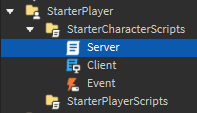# How come this script is not working

I am trying to make a script that anchors everything in the work space! This script works but is very glitchy and weird! for example i pressed e and everything anchored and somethimes when i press q the blocks unanchor but sometimes they dont but when you check the properties it seems to be unachored but is floating in the air

``````local Players = game:GetService("Players")
local LocalPlayer = Players.LocalPlayer
local UserInputService = game:GetService("UserInputService")

local function anchor(object)
object.Anchored = true
for _, child in pairs(object:GetChildren()) do
anchor(child)
end
end

local function unanchor(object)
object.Anchored = false
for _, child in pairs(object:GetChildren()) do
unanchor(child)
end
end

UserInputService.InputBegan:Connect(function(input)
if input.KeyCode == Enum.KeyCode.E then
local work = game.Workspace:GetChildren()
for i,v in pairs(work) do
if v:IsA("BasePart") and v.Name ~= "Baseplate" then
work[i].Anchored = true
end
if v:IsA("Model")then
local model = v:GetChildren()
for d,b in pairs(model) do
if b:IsA("BasePart") then
if b.Parent.Name ~= LocalPlayer.Name then
b.Anchored = true
end
end
end
end
end
elseif input.KeyCode == Enum.KeyCode.Q then
local work = game.Workspace:GetChildren()
for i,v in pairs(work) do
if v:IsA("BasePart") and v.Name ~= "Baseplate" then
work[i].Anchored = false
end
if v:IsA("Model")then
local model = v:GetChildren()
for d,b in pairs(model) do
if b:IsA("BasePart") then
b.Anchored = false
end
end
end
end
end
end)

``````

And i made this script in a local script if possible i would like to make this script server sidded but i dont know how

Locally unanchored objects won’t fall to the ground. It will move when you touched it. To make them anchored and unanchored server sided, you can use remote events.

Because you are utilizing a local script, only the client will be able to view the modifications. This explains why the parts’ attributes stated that they were unanchored.
I also suggest making a folder so you don’t have to check if you don’t want to accidentally anchor the player.
Here’s an example of how to make it server-side and more efficient.The scripts and events can be found in the StarterCharacterScripts and the folder is located in the workspace.

Our local script contains the following code:

``````local UserInputService = game:GetService("UserInputService")

local Event = script.Parent:WaitForChild('Event')

local function OnInputBegan(Input, gameProcessed)

if gameProcessed then return end

if Input.KeyCode == Enum.KeyCode.E then

Event:FireServer(true)

end

if Input.KeyCode == Enum.KeyCode.Q then

Event:FireServer(false)

end

end

UserInputService.InputBegan:Connect(OnInputBegan)
``````

And our server script contains:

``````local Event = script.Parent:WaitForChild('Event')

local function SetAnchor(player, isAnchored: boolean)

for _,v in pairs(workspace.Anchorable:GetDescendants()) do

if not v:IsA('BasePart') then continue end

v.Anchored = isAnchored

end

end

Event.OnServerEvent:Connect(SetAnchor)
``````

UserInputService.InputEnded:Connect(function(input)
if input.KeyCode == Enum.KeyCode.E then
local work = game.Workspace:GetChildren()
for i,v in pairs(work) do
if v:IsA(“BasePart”) and v.Name ~= “Baseplate” then
work[i].Anchored = true
end
if v:IsA(“Model”)then
local model = v:GetChildren()
for d,b in pairs(model) do
if b:IsA(“BasePart”) then
b.Anchored = true
end
end
end
end
elseif input.KeyCode == Enum.KeyCode.Q then
local work = game.Workspace:GetChildren()
for i,v in pairs(work) do
if v:IsA(“BasePart”) and v.Name ~= “Baseplate” then
work[i].Anchored = false
end
if v:IsA(“Model”)then
local model = v:GetChildren()
for d,b in pairs(model) do
if b:IsA(“BasePart”) then
b.Anchored = false
end
end
end
end
end
end)